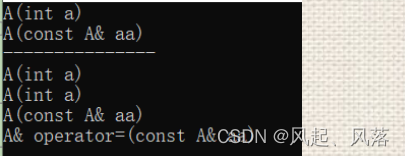# 类和对象(下）

C++基础知识

redux

## 1. 初始化列表

### 1. 概念

• 以一个冒号开始，接着是一个以逗号分隔的1数据成员列表，每个成员变量后面跟一个放在括号的初始值或表达式，即成员变量定义的地方

### 2. 用法

``````#include<iostream>
using namespace std;
class date
{
public:

private:
int _year;//声明
int _month;
int _day;
//const int x;//存在会报错
};
int main()
{
date d(2023, 2, 10);//定义
//const int a=0;
return 0;
}
``````
• const修饰的变量必须在定义时初始化，默认构造函数对内置类型不处理，对自定义类型调用它的默认构造函数，const int为内置类型，不会处理
• 正常来说，在date类实例化生成对象d时，是对象整体的定义，即对每个成员变量定义
• 但是像const修饰的变量必须在定义时初始化怎么办？
所以就有了初始化列表
• 那个对象调用构造函数，初始化列表是它所有成员变量定义的位置
• 不管是否显示在初始化列表写，编译器每个变量都会在初始化列表定义初始化

#### 成员变量出现随机值

``````#include<iostream>
using namespace std;
class date
{
public:
date()
:a(1)//使用初始化列表进行定义
,c(2)
{
}
private:
int a=2;//声明
int b=1;

int q;
};
int main()
{
date d;
return 0;
}
``````
• 可以在类中的成员变量声明处加入缺省值，若该变量在初始化列表中被定义，则该变量为被定义后的值，若在初始化列表中没有被定义，则该变量输出缺省值

WSA

• a=1,b=1,q=随机值

• 不管是否在初始化列表中写入全部的成员变量，都会全部调用一次

慢查询

• 例如 a，b，两者都有缺省值，在初始化列表中a被定义为1，则输出a=1，
b在初始化列表没有被定义,所以输出缺省值，b=1
q作为内置类型int，没有缺省值，也没有初始化列表定义，所以q为随机值

redis 复制集群搭建手册

### 3.注意事项

1.每个成员变量只能在初始化列表出现一次(初始化只能初始化一次）
2.类中包含 引用成员变量 const成员变量 自定义类型成员(没有默认构造函数) ，必须放在初始化列表位置进行初始化，(剩下的成员在初始化列表写不写都行，但是上述这三类就必须在列表写)

``````#include<iostream>
using namespace std;
class A
{
public:
//A() //不传参数为默认构造函数
//    :_a(1)//如果自定义类型的构造函数为这个 就不用在初始化列表初始化
//{

//}
A(int d)
:_a(d)
{

}
private:
int _a;
};
class date
{
public:
date()
:a(2)
,b(1)
,c(a)
,aa(5)
{

}
private:
int a = 1;//内置类型int
const int b = 2;//const
int& c;//引用
A aa;//自定义类型并且没有默认构造函数
};
int main()
{
//a=2 b=1 c=2 aa._a=5
date d;
return 0;
}
``````
• 因为构造函数对于内置类型不处理，对于自定义类型调用它的默认构造函数，
所以定义时必须初始化的包含自定义类型(不带默认构造函数)

3.成员变量在类中的声明次序就是在初始化列表中的初始化顺序，在初始化列表中的先后次序无关

sql优化

#### 例题

``````class A
{
public:
A(int a)
:_a1(a) //1
, _a2(_a1)
//随机值
{}
void Print() {
cout << _a1 << " " << _a2 << endl;
}
private:
int _a2;
int _a1;
};
int main() {
A aa(1);
aa.Print();// 1随机值
}
``````

gzip

## 2.explicit关键字

### 1.单参数的构造函数 (C++98) ，支持类型转换

``````#include<iostream>
using namespace std;
class A
{
public:
A(int n)//构造函数
:_a(n)
{
cout << "A(int n)" << endl;
}
A(A& d)//拷贝构造
{
cout << "A(A&d)" << endl;
}
private:
int _a;
};
int main()
{
A a(1);//构造函数     构造
A b = 1;//隐式类型转换 构造+拷贝构造->优化  构造
//int i = 1;
//double d = i;//隐式类型转换
return 0;
}
``````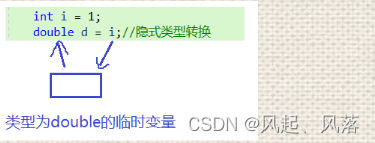• 是int对于double的类型转换，产生一个类型为double的临时变量，再将临时变量传给d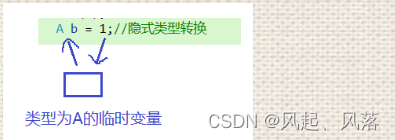• 内置类型int对于自定义类型A的类型转换,通过构造产生一个类型为A的临时变量，再通过拷贝构造传给b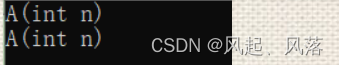#### 1. 验证临时变量的存在

``````#include<iostream>
using namespace std;
class A
{
public:
A(int n)
:_a(n)
{
cout << "A(int n)" << endl;
}
A(A& d)
{
cout << "A(A&d)" << endl;
}
private:
int _a;
};
int main()
{
//A& ret = 1;//错误
const A& ret = 1;
return 0;
}
``````
• A&ret=1会报错，而const A&ret=1却可以
• 因为通过构造生成一个类型为A的临时变量，而临时变量具有常性，ret为临时变量的别名， 将临时变量传过去，由const A类型到A类型会造成权限放大，所以要加const修饰ret，说明临时变量的存在

#### 2.关键字explicit的使用

``````#include<iostream>
using namespace std;
class A
{
public:
explicit A(int n)//构造
:_a(n)
{
cout << "A(int n)" << endl;
}
A(A& d)//拷贝构造
{
cout << "A(A&d)" << endl;
}
private:
int _a;
};
int main()
{
A a(1);//构造函数
A b = 1;//隐式类型转换 加入关键字explicit会报错
return 0;
}
``````
• 为了防止隐式类型转换发生，所以在构造函数前加入关键字explicit

### 2.多参数构造函数(C++11) 使用{ }支持类型转换

``````#include<iostream>
using namespace std;
class A
{
public:
explicit A(int n,int a)
:_a(n)
,_b(a)
{
cout << "A(int n,int a)" << endl;
}
A(A& d)
{
cout << "A(A&d)" << endl;
}
private:
int _a;
int _b;
};
int main()
{
//多参数的构造函数
A a(1, 2);
A a = { 1,2 };//隐式类型转换 加入关键字explicit就会报错
return 0;
}
``````

## 3.友元

### 1.友元函数

#### 2.实现cout功能

``````#include<iostream>
using namespace std;
class date
{
public:
date(int year, int month, int day)
{
_year = year;
_month = month;
_day = day;
}
void operator<<(ostream& out)
{
out << _year << "-" << _month <<"-"<< _day << endl;
}
private:
int _year;
int _month;
int _day;
};
int main()
{
date d1(2023, 2, 10);
//cout << d1; 我们想实现成的
d1 << cout; //实际在类中生成的
return 0;
}
``````

cout<<d1等价于cout.operator<<(d1)，若为cout<<d1，就会将cout作为左操作数，
cout是标准库std命名里面一个ostream类型的全局对象，与this指针类型不符，所以在类中只能写成 d1<<cout

WebSocket使用案例

``````#include<iostream>
using namespace std;
class date
{
public:
friend void operator<<(ostream& out, date& d);//firend+函数定义 说明函数为类的友元函数
date(int year, int month, int day)
{
_year = year;
_month = month;
_day = day;
}
private:
int _year;
int _month;
int _day;
};
void operator<<(ostream& out, date&d)
{
out << d._year << "-" << d._month << "-" << d._day << endl;
}
int main()
{
date d1(2023, 2, 10);
cout << d1;
return 0;
}
``````

#### 3.实现cin的功能

``````#include<iostream>
using namespace std;
class date
{
public:
friend void operator>>(istream& in, date& d);
private:
int _year;
int _month;
int _day;
};
void operator>>(istream& in, date& d)
{
in >> d._year >> d._month >> d._day;
}
int main()
{
date d1;
cin >> d1;
return 0;
}
``````

cin是标准库std命名里面一个istream类型的全局对象，

#### 4.说明

1.友元函数可以访问类的私有和保护成员，但是不是类的成员函数
2.友元函数不能用const修饰
3.友元函数可以在类的定义的任何地方声明，不受访问限定符限制

### 2.友元类

``````class Time
{
public:
friend class date;//日期类是时间类的友元
Time(int hours=0,int minute=0,int second=0)
{
_hour = hours;
_minute = minute;
_second = second;
}
private:
int _hour;
int _minute;
int _second;
};
class date
{
public:
date(int year=1, int month=1, int day=1)
{
_year = year;
_month = month;
_day = day;
}
void print(int hour,int minute,int second)
{
//直接调用时间类的私有成员变量
_t._hour = hour;
_t._minute = minute;
_t._second = second;
}
private:
int _year;
int _month;
int _day;
Time _t;
};

``````

TI

#### 说明

1.友元是单向的，不具有交换性
2.友元不能传递，如果C是B的友元，B是A的友元，不能说明C是A的友元

stylegan

## 4.static 成员

arrays

### 2.实现一个类，计算类中创建了多少个对象

``````#include<iostream>
using namespace std;
class A
{
public:
A(int a=0)
{
n++;
}
A(A& d)
{
n++;
}
static int getn()//静态成员函数没有this指针
{
return n;
}
private:
//不属于某个对象，属于所有对象，属于整个类
static int n;//静态成员变量声明
};
int A::n = 0;//静态成员变量定义
int main()
{
A a;//构造
A b;//构造
A c(a);//拷贝构造
A d(b);//拷贝构造
cout << A::getn() << endl;//4
return 0;
}
``````

openid

### 3. 特性

• 1.静态成员为所有类对象所共享，不属于某个具体的对象，存放在静态区
static修饰的成员变量 不属于某个对象，属于所有对象，属于整个类

智能车大赛

• 2. 静态成员变量必须在类外定义，定义时不添加static关键字，类中只是声明
静态成员变量属于共有的，不能在初始化列表初始化，所以只能在类外初始化

rsync

• 3.静态成员函数没有隐藏的this指针，不能访问任何非静态成员

微信小程序支付

• 4.静态成员也是类的成员，受public、protected、private 访问限定符的限制
若在类外直接使用，对象调用处于类中私有的成员变量

Java毕设项目实战

## 5. 匿名对象

``````#include<iostream>
using namespace std;
class date
{
public:
date()
{
cout << "date()" << endl;
}
~date()
{
cout << "~date()" << endl;
}
void print()
{
cout << "print" << endl;
}
private:
int _year;
int _month;
int _day;
};
int main()
{
date();//匿名对象，生命周期只在第一行
date().print();
return 0;
}
``````

#### 匿名对象的返回

``````#include<iostream>
using namespace std;
class date
{
public:
void print()
{
cout << "print" << endl;
}
int  sum(int n)
{
return n;
}
private:
int _year;
int _month;
int _day;
};
class A
{
public:
A(int n)
{

}
};
A fun()
{
int n = 0;
cin >> n;
int ret = date().sum(n);
//A d=ret; //正常来说创建一个对象接收 ，在返回对象
//return d;
return A(ret);//直接返回匿名对象
}
int main()
{
date();//匿名对象，生命周期只在第一行

return 0;
}
``````
• 正常来说，需要创建一个A类型对象通过隐式类型转换接收ret，在返回对象 或者直接返回匿名对象 A(ret)

## 6.内部类

``````#include<iostream>
using namespace std;
class A
{
private:
int n;
public:
class B
{
public:
void print()
{

}
private:
int b;
};
};
int main()
{
cout << sizeof(A) << endl;//4
A::B b;//必须通过A类，然后才能访问B类创建对象b
return 0;
}
``````

### 内部类是外部类的友元

``````class A
{
public:
class B//B是A的友元
{
public:
void print(A&d)
{
//在B类中可以调用A的私有成员变量
cout << d.n << endl;
}
private:
int b;

};
private:
int n;
};
int main()
{
cout << sizeof(A) << endl;//4
return 0;
}
``````
• 可以在内部类B中调用外部类A的私有成员变量n

## 7.拷贝对象时的一些编译器优化

``````class A
{
public:
A(int a = 0)
:_a(a)
{
cout << "A(int a)" << endl;
}
private:
int _a;
};
int main()
{
A aa=1;//构造函数+拷贝构造 优化为 构造
return 0;
}
``````

### 1.传值传参

``````class A
{
public:
A(int a = 0)
:_a(a)
{
cout << "A(int a)" << endl;
}
private:
int _a;
};
void func(A a)
{

}
int main()
{
func(2);//构造+拷贝构造 优化-> 构造
return 0;
}
``````
• 这里的传值传参func(2)可以看作是在一个表达式中，将内置类型int的2传给 自定义类型A的a，发生隐式类型转换
• 编译器 把构造函数+拷贝构造 优化为构造

### 2.引用传参

``````class A
{
public:
A(int a = 0)
:_a(a)
{
cout << "A(int a)" << endl;
}
private:
int _a;
};

void func2(const A& a)//必须使用const修饰，临时变量具有常性
{

}
int main()
{
func2(2);//无优化

return 0;
}
``````
• A&a=2 这样写会报错，而加上const 修饰变成 const A&a=2就通过了
说明存在临时变量，而临时变量具有常性，a作为临时变量的别名，要加上const保持权限一致
• 无优化，必须存在临时变量

### 3.传值返回

``````class A
{
public:
A(int a = 0)
:_a(a)
{
cout << "A(int a)" << endl;
}
A(const A& aa)
:_a(aa._a)
{
cout << "A(const A& aa)" << endl;
}
private:
int _a;
};

A func3()
{
A a;//构造
return a;
//拷贝构造
}
int main()
{
func3();//构造+拷贝构造 无优化
return 0;
}
``````

return a返回时，因为传值返回，所以会拷贝构造一个临时变量

``````#include<iostream>
using namespace std;

class A
{
public:
A(int a = 0)
:_a(a)
{
cout << "A(int a)" << endl;
}
A(const A& aa)
:_a(aa._a)
{
cout << "A(const A& aa)" << endl;
}
private:
int _a;
};

A func3()
{
A a;
return a;
}
int main()
{
A b=func3();
return 0;
}
``````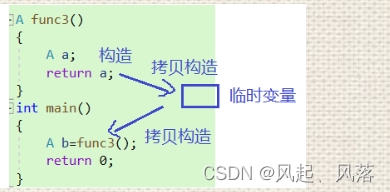• retun a返回通过拷贝构造生成一个临时变量，再把临时比变量拷贝构传给b 正常来说是进行 一次构造、两次拷贝构造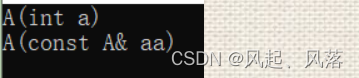### 4.匿名对象的传值返回

``````
class A
{
public:
A(int a = 0)
:_a(a)
{
cout << "A(int a)" << endl;
}
A(const A& aa)
:_a(aa._a)
{
cout << "A(const A& aa)" << endl;
}
private:
int _a;
};

A func4()
{
return A();
}
int main()
{
A b=func4();//构造+拷贝构造+拷贝构造  优化成 构造
return 0;
}

``````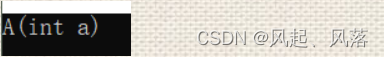### 5.两种习惯之间的差异

``````class A
{
public:
A(int a = 0)
:_a(a)
{
cout << "A(int a)" << endl;
}
A(const A& aa)
:_a(aa._a)
{
cout << "A(const A& aa)" << endl;
}
A& operator=(const A& aa)
{
cout << "A& operator=(const A& aa)" << endl;
if (this != &aa)
{
_a = aa._a;
}
return *this;
}
private:
int _a;
};

A func4()
{
A a;
return a;
}
int main()
{
A c = func4();//构造+拷贝构造+拷贝构造 优化->构造+拷贝构造
cout << "---------------" << endl;
A b;//构造
b = func4();//构造+拷贝构造+赋值  无优化
return 0;
}
``````